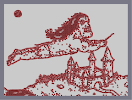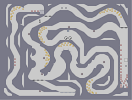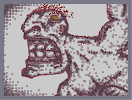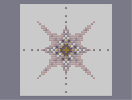### Harry's Flying RevengeHover over the thumbnail for a full-size version.

Author espada777777 author:espada777777 harry harryandthepotter harrypotter n-art rated 2008-03-28 2008-03-28 4 by 21 people. \$Harry's Flying Revenge#espada777777#none#00000000000000000000000000000000000000000000000000000000000000000000000000000000000000000000000000000000000000000000000000000000000000000000000000000000000000000000000000000000000000000000000000000000000000000000000000000000000000000000000000000000000000000000000000000000000000000000000000000000000000000000000000000000000000000000000000000000000000000000000000000000000000000000000000000000000000000000000000000000000000000000000000000000000000000000000000000000000000000000000000000000000000000000000000000000000000000000000000000000000000000000000000000000000000000000000000000000000000000000000000000000000000000000000000000000000000000000000000000000000000000000000000000000000000000000000000000000000000000|12^440,235!12^442,234!12^448,231!12^453,229!12^458,229!12^464,229!12^468,229!12^471,229!12^474,231!12^478,232!12^481,235!12^482,237!12^482,237!12^484,241!12^486,246!12^486,248!12^487,251!12^489,255!12^489,255!12^488,245!12^488,245!12^489,245!12^492,244!12^498,244!12^501,243!12^507,243!12^509,245!12^514,247!12^518,248!12^522,251!12^525,254!12^529,258!12^531,260!12^532,264!12^533,268!12^533,268!12^533,268!12^533,270!12^531,263!12^534,261!12^533,272!12^533,276!12^533,276!12^533,277!12^533,279!12^598,253!12^600,253!12^602,252!12^604,249!12^606,249!12^609,248!12^593,255!12^610,247!12^615,245!12^616,245!12^620,245!12^624,245!12^625,246!12^628,246!12^633,247!12^637,247!12^640,248!12^640,250!12^641,250!12^641,254!12^638,257!12^631,256!12^629,256!12^626,256!12^631,257!12^634,257!12^638,257!12^639,259!12^639,259!12^638,263!12^636,264!12^633,265!12^630,266!12^628,266!12^624,266!12^636,267!12^635,271!12^634,273!12^630,274!12^628,275!12^625,275!12^624,275!12^621,275!12^628,278!12^622,277!12^619,279!12^616,279!12^629,278!12^620,275!12^615,275!12^611,280!12^609,279!12^607,279!12^603,279!12^603,279!12^604,278!12^601,282!12^599,284!12^596,287!12^593,290!12^590,292!12^587,295!12^581,298!12^576,300!12^572,301!12^564,304!12^563,304!12^557,305!12^554,306!12^552,306!12^595,256!12^592,257!12^588,258!12^586,259!12^584,259!12^581,259!12^578,259!12^574,259!12^572,259!12^568,259!12^567,259!12^564,260!12^562,261!12^559,262!12^557,263!12^554,263!12^551,263!12^547,264!12^546,265!12^543,266!12^542,267!12^540,269!12^535,271!12^535,271!12^552,306!12^549,306!12^548,306!12^544,308!12^550,306!12^546,310!12^543,312!12^540,313!12^532,313!12^532,313!12^527,311!12^526,309!12^524,309!12^524,309!12^524,305!12^523,307!12^522,308!12^521,309!12^517,309!12^517,309!12^512,312!12^512,312!12^511,312!12^506,312!12^504,312!12^501,312!12^500,312!12^499,312!12^497,311!12^495,310!12^480,307!12^494,309!12^489,309!12^487,308!12^483,308!12^482,306!12^481,305!12^480,302!12^478,300!12^477,299!12^475,296!12^474,293!12^472,298!12^472,298!12^470,300!12^469,302!12^467,302!12^463,302!12^462,302!12^471,294!12^471,294!12^469,291!12^465,290!12^625,244!12^627,243!12^632,238!12^637,235!12^639,235!12^627,247!12^632,240!12^636,238!12^639,233!12^647,227!12^634,234!12^634,235!12^641,231!12^643,229!12^645,227!12^647,226!12^652,223!12^655,220!12^659,218!12^661,217!12^664,214!12^666,212!12^667,210!12^669,209!12^672,206!12^672,205!12^674,203!12^676,204!12^681,202!12^682,202!12^637,238!12^636,240!12^637,240!12^643,232!12^646,230!12^651,226!12^652,226!12^639,235!12^637,237!12^643,233!12^649,228!12^653,222!12^655,222!12^662,218!12^547,208!12^547,212!12^547,214!12^547,218!12^546,219!12^542,221!12^540,222!12^536,222!12^550,215!12^550,215!12^552,214!12^553,212!12^553,211!12^554,208!12^554,206!12^554,203!12^554,201!12^554,198!12^552,194!12^551,193!12^548,189!12^548,189!12^545,188!12^539,190!12^535,190!12^531,191!12^530,191!12^527,192!12^527,192!12^524,192!12^520,192!12^518,190!12^515,190!12^512,188!12^510,184!12^509,180!12^509,179!12^507,174!12^507,172!12^507,172!12^511,171!12^516,170!12^518,170!12^522,170!12^525,169!12^529,168!12^531,167!12^535,167!12^538,167!12^541,167!12^543,167!12^543,168!12^543,168!12^543,171!12^543,173!12^543,176!12^545,178!12^546,183!12^546,184!12^515,182!12^516,183!12^518,183!12^521,182!12^523,181!12^527,181!12^527,181!12^530,181!12^534,181!12^535,180!12^538,180!12^540,180!12^541,180!12^533,172!12^533,176!12^533,178!12^534,181!12^534,184!12^518,174!12^520,182!12^520,185!12^521,186!12^521,190!12^526,135!12^527,139!12^527,141!12^531,146!12^536,147!12^538,151!12^539,154!12^536,152!12^531,146!12^530,139!12^528,138!12^538,159!12^536,158!12^531,159!12^527,159!12^525,160!12^522,161!12^515,152!12^512,156!12^512,159!12^512,162!12^511,160!12^507,176!12^507,178!12^506,181!12^506,184!12^506,188!12^506,189!12^511,191!12^513,190!12^516,190!12^517,190!12^512,190!12^493,120!12^493,120!12^489,121!12^486,124!12^484,128!12^483,133!12^483,136!12^483,139!12^486,143!12^489,144!12^492,144!12^496,144!12^498,144!12^501,143!12^502,142!12^504,139!12^505,137!12^506,135!12^506,134!12^506,130!12^505,127!12^505,126!12^504,124!12^502,122!12^500,122!12^499,120!12^498,120!12^496,136!12^500,131!12^500,131!12^499,131!12^494,130!12^493,129!12^489,127!12^492,137!12^511,131!12^510,131!12^514,129!12^518,129!12^521,127!12^523,127!12^525,128!12^526,128!12^528,129!12^533,119!12^529,124!12^529,128!12^529,133!12^529,135!12^532,136!12^534,132!12^535,127!12^535,121!12^535,117!12^541,165!12^540,159!12^540,159!12^499,167!12^498,168!12^497,170!12^497,171!12^500,197!12^501,199!12^502,199!12^504,200!12^515,101!12^513,101!12^510,105!12^510,107!12^513,108!12^516,109!12^516,112!12^515,116!12^515,117!12^501,97!12^499,98!12^497,98!12^495,98!12^491,98!12^488,99!12^484,101!12^482,103!12^478,106!12^477,107!12^476,108!12^472,113!12^471,115!12^470,116!12^468,119!12^468,120!12^465,125!12^465,125!12^533,223!12^531,225!12^528,225!12^526,225!12^524,225!12^524,225!12^523,225!12^521,225!12^518,226!12^514,225!12^510,224!12^506,224!12^502,223!12^499,223!12^496,221!12^494,220!12^491,217!12^489,215!12^486,211!12^485,207!12^484,203!12^482,198!12^482,193!12^481,191!12^479,185!12^497,224!12^497,224!12^495,226!12^493,228!12^493,228!12^472,144!12^470,144!12^468,145!12^465,147!12^464,148!12^464,149!12^464,155!12^465,159!12^468,161!12^469,165!12^472,166!12^475,164!12^476,163!12^471,153!12^470,159!12^508,95!12^504,95!12^528,101!12^526,99!12^525,97!12^522,95!12^520,93!12^516,90!12^512,88!12^509,88!12^507,87!12^512,96!12^519,96!12^521,98!12^524,99!12^527,103!12^527,106!12^529,108!12^530,113!12^530,116!12^530,117!12^510,87!12^509,87!12^503,84!12^501,84!12^499,84!12^495,84!12^494,83!12^492,83!12^484,83!12^483,84!12^482,84!12^479,84!12^467,85!12^484,84!12^476,85!12^472,84!12^468,84!12^467,84!12^463,85!12^462,86!12^455,88!12^454,89!12^453,90!12^449,95!12^447,97!12^443,102!12^441,104!12^436,108!12^434,110!12^425,117!12^423,120!12^421,122!12^420,123!12^419,126!12^414,132!12^410,136!12^408,138!12^407,139!12^406,141!12^404,143!12^398,149!12^397,149!12^389,152!12^387,152!12^384,153!12^380,155!12^377,159!12^373,162!12^369,166!12^365,170!12^359,174!12^357,175!12^352,176!12^351,176!12^343,181!12^342,182!12^338,187!12^337,188!12^336,190!12^332,193!12^330,194!12^327,197!12^323,198!12^316,200!12^310,200!12^308,200!12^304,200!12^297,200!12^293,200!12^291,199!12^287,198!12^287,198!12^286,197!12^282,196!12^282,196!12^285,203!12^289,208!12^285,200!12^289,205!12^290,211!12^292,215!12^293,219!12^296,221!12^301,223!12^302,225!12^304,228!12^310,230!12^315,232!12^317,233!12^321,234!12^323,234!12^326,234!12^328,234!12^332,234!12^343,233!12^346,232!12^346,232!12^340,235!12^347,234!12^350,234!12^356,234!12^360,235!12^363,235!12^368,235!12^372,235!12^377,234!12^388,229!12^388,229!12^387,230!12^384,232!12^391,227!12^393,225!12^396,222!12^397,220!12^399,217!12^401,215!12^403,210!12^405,207!12^407,206!12^409,205!12^412,204!12^416,203!12^420,202!12^423,201!12^426,198!12^427,195!12^429,194!12^431,194!12^430,194!12^431,192!12^434,192!12^437,190!12^441,186!12^444,183!12^446,180!12^448,176!12^449,175!12^450,174!12^453,171!12^455,169!12^456,167!12^460,165!12^462,165!12^464,164!12^472,117!12^470,121!12^469,126!12^466,132!12^466,135!12^466,138!12^465,142!12^472,118!12^474,124!12^474,126!12^476,110!12^478,113!12^483,102!12^487,104!12^495,100!12^499,101!12^434,108!12^433,109!12^432,109!12^430,111!12^427,113!12^423,113!12^418,113!12^411,113!12^410,112!12^410,110!12^409,111!12^414,116!12^422,115!12^432,109!12^440,98!12^445,94!12^442,95!12^437,100!12^428,100!12^430,98!12^440,94!12^448,89!12^456,87!12^464,84!12^476,83!12^472,83!12^467,82!12^457,82!12^452,82!12^449,84!12^463,81!12^482,80!12^492,80!12^481,77!12^478,77!12^485,78!12^493,82!12^502,86!12^509,86!12^514,90!12^414,130!12^410,133!12^405,132!12^400,132!12^397,131!12^394,129!12^393,130!12^401,132!12^405,132!12^402,141!12^400,143!12^390,146!12^386,147!12^377,150!12^376,150!12^370,149!12^370,149!12^378,147!12^385,146!12^392,146!12^393,146!12^399,143!12^394,143!12^388,140!12^383,138!12^384,138!12^398,139!12^404,135!12^412,131!12^416,124!12^418,120!12^409,129!12^408,130!12^405,130!12^378,159!12^374,160!12^371,163!12^364,166!12^361,167!12^355,168!12^352,168!12^349,166!12^354,166!12^357,167!12^354,171!12^353,173!12^349,177!12^346,179!12^338,179!12^335,179!12^332,179!12^331,178!12^336,181!12^340,185!12^335,191!12^333,192!12^322,194!12^319,194!12^309,194!12^303,192!12^301,192!12^304,193!12^310,195!12^322,195!12^323,195!12^309,193!12^303,192!12^300,185!12^300,179!12^300,174!12^308,174!12^309,176!12^312,180!12^312,182!12^309,175!12^303,174!12^302,176!12^301,181!12^307,188!12^312,192!12^319,195!12^321,196!12^293,202!12^288,200!12^286,190!12^284,188!12^283,179!12^286,179!12^290,181!12^291,184!12^291,184!12^288,178!12^285,179!12^284,192!12^292,195!12^292,200!12^288,201!12^281,195!12^276,191!12^274,189!12^274,184!12^274,192!12^279,196!12^284,201!12^287,210!12^288,213!12^286,213!12^281,210!12^279,207!12^278,212!12^286,215!12^290,215!12^298,221!12^297,221!12^295,223!12^290,223!12^286,224!12^280,223!12^280,223!12^292,224!12^313,228!12^311,231!12^307,233!12^306,233!12^328,231!12^328,235!12^322,241!12^317,243!12^316,243!12^330,238!12^337,233!12^339,233!12^337,235!12^336,241!12^333,246!12^335,242!12^340,228!12^347,228!12^363,227!12^359,234!12^359,236!12^353,244!12^357,244!12^363,235!12^373,235!12^376,234!12^377,243!12^376,243!12^383,235!12^385,233!12^392,225!12^400,218!12^398,229!12^400,231!12^400,220!12^401,214!12^404,211!12^406,210!12^411,208!12^419,210!12^418,212!12^417,208!12^419,206!12^421,203!12^426,201!12^427,198!12^430,197!12^434,194!12^434,193!12^432,195!12^432,200!12^433,201!12^435,200!12^435,192!12^439,184!12^443,180!12^446,178!12^451,174!12^462,166!12^462,167!12^459,172!12^459,173!12^459,177!12^458,180!12^459,172!12^460,168!12^465,169!12^467,175!12^470,177!12^471,177!12^465,131!12^461,137!12^464,119!12^456,96!12^468,96!12^480,96!12^468,108!12^456,108!12^444,108!12^456,120!12^432,120!12^420,132!12^432,132!12^444,132!12^456,132!12^456,144!12^444,144!12^420,144!12^408,144!12^456,156!12^444,156!12^432,156!12^420,156!12^408,156!12^396,156!12^384,156!12^372,168!12^384,168!12^396,168!12^408,168!12^420,168!12^432,168!12^444,168!12^456,168!12^432,180!12^420,180!12^408,180!12^396,180!12^384,180!12^360,180!12^372,180!12^348,180!12^336,192!12^348,192!12^372,192!12^384,192!12^396,192!12^408,192!12^420,192!12^432,192!12^408,204!12^396,204!12^384,204!12^372,204!12^360,204!12^492,84!12^480,84!12^348,204!12^336,204!12^324,204!12^312,204!12^300,204!12^288,204!12^300,216!12^312,216!12^324,216!12^336,216!12^348,216!12^360,216!12^372,216!12^384,216!12^396,216!12^384,228!12^372,228!12^360,228!12^348,228!12^336,228!12^324,228!12^312,228!12^498,90!12^492,90!12^504,90!12^486,90!12^480,90!12^474,90!12^468,90!12^462,90!12^492,96!12^486,96!12^474,96!12^462,96!12^450,102!12^456,102!12^462,102!12^468,102!12^474,102!12^462,108!12^444,108!12^450,108!12^432,114!12^438,114!12^444,114!12^456,114!12^462,114!12^462,120!12^450,120!12^438,120!12^426,120!12^426,126!12^432,126!12^444,126!12^450,126!12^456,126!12^450,132!12^426,132!12^414,138!12^420,138!12^426,138!12^432,138!12^444,138!12^450,138!12^456,138!12^462,150!12^456,150!12^444,150!12^450,150!12^444,144!12^450,144!12^438,144!12^426,144!12^414,144!12^438,150!12^432,150!12^426,150!12^420,150!12^408,150!12^414,150!12^402,150!12^390,156!12^402,156!12^414,156!12^426,156!12^438,156!12^450,156!12^456,162!12^444,162!12^438,174!12^438,162!12^432,162!12^450,162!12^462,126!12^462,132!12^426,162!12^420,162!12^414,162!12^402,162!12^408,162!12^396,162!12^390,162!12^384,162!12^378,168!12^390,168!12^402,168!12^414,168!12^426,168!12^438,168!12^450,168!12^444,174!12^438,180!12^432,186!12^426,186!12^426,192!12^420,198!12^414,198!12^432,174!12^426,174!12^414,174!12^420,174!12^408,174!12^402,174!12^396,174!12^390,174!12^384,174!12^378,174!12^372,174!12^366,174!12^360,174!12^426,180!12^462,144!12^462,156!12^468,114!12^438,108!12^378,162!12^390,150!12^414,180!12^402,180!12^390,180!12^366,180!12^354,180!12^348,186!12^354,186!12^366,186!12^360,186!12^378,186!12^384,186!12^390,186!12^396,186!12^402,186!12^408,186!12^414,186!12^420,186!12^414,192!12^402,192!12^390,192!12^378,192!12^354,192!12^342,192!12^342,186!12^408,198!12^396,198!12^402,198!12^390,198!12^384,198!12^378,198!12^372,198!12^366,198!12^360,198!12^354,198!12^348,198!12^342,198!12^336,198!12^330,198!12^294,204!12^306,204!12^318,204!12^330,204!12^342,204!12^354,204!12^366,204!12^378,204!12^390,204!12^402,204!12^396,210!12^390,210!12^384,210!12^372,210!12^378,210!12^360,210!12^366,210!12^354,210!12^348,210!12^342,210!12^336,210!12^330,210!12^318,210!12^324,210!12^312,210!12^306,210!12^300,210!12^390,216!12^378,216!12^390,222!12^384,222!12^378,222!12^372,222!12^372,228!12^378,228!12^366,228!12^354,228!12^336,228!12^330,228!12^318,228!12^306,228!12^306,222!12^306,216!12^294,210!12^312,216!12^318,222!12^318,222!12^312,222!12^318,216!12^330,222!12^324,222!12^342,216!12^354,216!12^366,222!12^455,199!12^454,200!12^453,202!12^450,207!12^447,210!12^443,217!12^442,220!12^439,224!12^473,217!12^473,219!12^472,222!12^472,222!12^472,225!12^472,225!12^494,230!12^493,232!12^493,236!12^493,238!12^493,240!12^417,274!12^417,278!12^417,282!12^423,286!12^426,288!12^429,292!12^432,293!12^437,296!12^465,304!12^465,307!12^465,307!12^457,312!12^453,313!12^451,313!12^448,313!12^444,314!12^441,315!12^438,315!12^435,316!12^432,317!12^428,318!12^426,319!12^423,320!12^421,320!12^418,321!12^415,321!12^410,323!12^404,325!12^400,326!12^395,327!12^390,327!12^384,327!12^384,327!12^379,326!12^377,315!12^376,320!12^378,323!12^379,324!12^383,326!12^379,326!12^377,328!12^375,329!12^373,329!12^372,329!12^370,331!12^367,331!12^365,330!12^362,327!12^361,325!12^358,320!12^358,322!12^364,332!12^360,334!12^358,334!12^355,334!12^352,334!12^349,334!12^345,334!12^343,334!12^342,329!12^341,325!12^342,334!12^342,333!12^339,335!12^334,335!12^330,335!12^326,335!12^326,335!12^373,306!12^362,307!12^348,311!12^337,314!12^360,306!12^372,305!12^382,305!12^391,310!12^397,314!12^389,310!12^379,305!12^368,307!12^355,310!12^347,312!12^342,312!12^341,312!12^391,310!12^397,315!12^429,315!12^425,310!12^425,310!12^424,305!12^423,304!12^420,300!12^420,300!12^423,309!12^426,315!12^427,316!12^425,315!12^427,319!12^430,324!12^431,324!12^435,322!12^440,320!12^431,311!12^439,309!12^441,321!12^446,319!12^451,317!12^455,316!12^458,315!12^462,312!12^464,309!12^464,302!12^423,325!12^326,336!12^324,336!12^322,336!12^318,337!12^317,338!12^312,338!12^308,338!12^305,337!12^302,333!12^300,331!12^299,328!12^299,325!12^298,320!12^298,318!12^298,313!12^297,305!12^296,302!12^295,296!12^294,293!12^293,290!12^292,286!12^291,283!12^289,280!12^288,278!12^287,275!12^287,272!12^286,269!12^286,265!12^286,263!12^286,261!12^286,260!12^289,257!12^291,256!12^294,254!12^295,253!12^298,253!12^303,253!12^306,252!12^307,251!12^309,250!12^312,249!12^314,247!12^316,246!12^320,244!12^323,244!12^325,243!12^327,243!12^327,242!12^327,242!12^330,242!12^331,242!12^291,252!12^285,254!12^282,254!12^278,255!12^275,256!12^272,257!12^270,257!12^266,259!12^262,259!12^262,259!12^497,230!12^502,230!12^504,231!12^506,232!12^512,232!12^514,232!12^518,234!12^522,236!12^526,236!12^529,238!12^533,241!12^536,243!12^538,245!12^539,249!12^540,251!12^540,251!12^541,245!12^541,245!12^544,244!12^549,243!12^553,242!12^557,242!12^564,241!12^566,241!12^572,242!12^576,242!12^579,242!12^585,243!12^587,244!12^589,245!12^591,246!12^593,247!12^594,244!12^597,244!12^598,244!12^602,242!12^604,241!12^608,241!12^612,241!12^615,240!12^616,240!12^616,240!12^619,240!12^621,241!12^308,339!12^303,340!12^301,341!12^299,343!12^297,343!12^294,344!12^293,344!12^288,345!12^284,346!12^280,346!12^278,346!12^277,346!12^275,347!12^271,347!12^269,347!12^267,347!12^266,347!12^263,347!12^261,348!12^259,348!12^254,349!12^248,349!12^245,349!12^242,349!12^238,349!12^235,350!12^234,350!12^230,350!12^231,350!12^234,345!12^239,340!12^242,338!12^243,335!12^246,332!12^248,331!12^247,331!12^242,330!12^239,330!12^234,330!12^233,330!12^227,330!12^225,330!12^222,330!12^219,330!12^218,330!12^221,326!12^225,325!12^227,322!12^230,320!12^233,318!12^233,317!12^233,316!12^225,314!12^225,314!12^227,311!12^230,309!12^234,307!12^236,305!12^239,303!12^240,302!12^241,301!12^238,299!12^233,298!12^230,297!12^228,297!12^227,297!12^225,296!12^219,295!12^219,295!12^215,295!12^215,294!12^218,292!12^220,291!12^223,289!12^225,288!12^229,286!12^232,285!12^236,282!12^237,282!12^238,281!12^239,281!12^241,280!12^244,276!12^253,270!12^264,267!12^258,261!12^254,261!12^251,261!12^247,263!12^244,265!12^242,266!12^240,267!12^237,267!12^235,267!12^231,267!12^229,268!12^225,268!12^222,268!12^219,268!12^216,268!12^213,268!12^210,268!12^210,268!12^210,268!12^212,268!12^215,269!12^219,270!12^223,272!12^224,276!12^226,278!12^228,279!12^230,280!12^231,282!12^232,282!12^210,295!12^243,277!12^245,276!12^250,274!12^254,273!12^258,271!12^261,270!12^262,269!12^268,269!12^268,268!12^281,265!12^281,265!12^279,266!12^216,299!12^214,299!12^209,301!12^207,302!12^205,303!12^203,304!12^201,305!12^199,306!12^220,300!12^199,306!12^195,307!12^192,309!12^190,311!12^188,313!12^188,313!12^188,314!12^188,315!12^188,315!12^193,306!12^192,306!12^187,308!12^182,308!12^179,308!12^174,308!12^172,308!12^170,309!12^167,309!12^163,310!12^159,310!12^156,311!12^151,313!12^147,314!12^144,316!12^142,317!12^140,318!12^137,319!12^133,321!12^130,323!12^126,325!12^125,325!12^125,325!12^228,347!12^223,348!12^220,349!12^217,350!12^215,350!12^213,351!12^210,351!12^208,352!12^205,352!12^200,353!12^198,353!12^195,354!12^189,354!12^186,354!12^184,351!12^183,348!12^182,346!12^182,346!12^185,352!12^185,352!12^181,354!12^179,354!12^175,355!12^175,355!12^172,356!12^163,358!12^160,358!12^155,357!12^151,357!12^147,357!12^143,357!12^138,356!12^134,356!12^133,356!12^133,356!12^146,357!12^153,357!12^163,358!12^168,358!12^205,344!12^205,348!12^203,352!12^203,352!12^206,349!12^197,337!12^131,355!12^127,355!12^126,355!12^125,355!12^122,356!12^122,356!12^118,356!12^222,280!12^219,281!12^218,282!12^215,283!12^212,283!12^208,284!12^207,284!12^205,284!12^202,285!12^199,286!12^196,286!12^196,287!12^195,288!12^193,288!12^192,291!12^190,293!12^189,295!12^188,296!12^187,297!12^190,288!12^189,288!12^187,288!12^183,288!12^181,288!12^179,289!12^173,289!12^173,289!12^171,290!12^169,290!12^166,290!12^165,290!12^162,290!12^160,291!12^156,292!12^154,293!12^151,294!12^148,295!12^144,296!12^139,298!12^137,299!12^134,299!12^131,301!12^130,301!12^128,301!12^308,336!12^308,336!12^305,336!12^304,332!12^303,328!12^303,325!12^306,253!12^304,255!12^301,258!12^300,259!12^299,263!12^299,263!12^122,324!12^122,324!12^121,322!12^125,323!12^122,323!12^119,324!12^117,324!12^113,324!12^110,324!12^110,323!12^119,321!12^114,321!12^111,321!12^108,319!12^108,319!12^106,319!12^102,320!12^100,322!12^97,324!12^95,326!12^93,328!12^93,329!12^91,331!12^88,334!12^86,339!12^85,342!12^82,347!12^81,349!12^77,355!12^74,357!12^70,359!12^67,361!12^62,364!12^60,365!12^57,368!12^116,358!12^115,360!12^116,360!12^114,360!12^112,361!12^110,362!12^108,363!12^107,364!12^102,367!12^101,367!12^98,369!12^95,370!12^94,371!12^91,373!12^89,374!12^87,374!12^84,375!12^82,378!12^81,378!12^78,380!12^77,381!12^76,381!12^73,382!12^72,382!12^68,382!12^66,383!12^65,384!12^61,386!12^59,387!12^57,389!12^57,389!12^55,391!12^53,392!12^52,392!12^50,392!12^47,390!12^46,387!12^46,386!12^48,383!12^50,380!12^50,380!12^52,377!12^54,375!12^55,372!12^55,370!12^52,371!12^48,371!12^45,375!12^43,377!12^43,380!12^43,380!12^46,380!12^52,369!12^47,369!12^49,368!12^58,365!12^58,365!12^45,371!12^52,369!12^54,367!12^72,354!12^74,352!12^77,347!12^80,345!12^83,342!12^83,341!12^83,337!12^83,336!12^84,334!12^85,332!12^86,331!12^88,326!12^90,325!12^94,323!12^94,321!12^99,320!12^103,319!12^50,363!12^60,361!12^66,357!12^54,363!12^54,363!12^44,366!12^42,367!12^120,354!12^121,352!12^122,348!12^122,348!12^65,382!12^65,381!12^65,379!12^127,302!12^126,302!12^125,302!12^122,302!12^119,301!12^112,300!12^112,300!12^109,300!12^106,301!12^90,311!12^86,313!12^83,315!12^81,317!12^79,319!12^79,319!12^77,321!12^75,323!12^73,325!12^72,326!12^71,328!12^68,330!12^65,330!12^64,331!12^62,336!12^61,338!12^60,340!12^57,342!12^56,343!12^55,344!12^54,344!12^52,346!12^51,347!12^51,348!12^51,351!12^51,352!12^52,353!12^55,354!12^56,354!12^59,353!12^60,352!12^62,351!12^64,350!12^67,349!12^69,348!12^71,348!12^61,337!12^65,337!12^69,333!12^69,332!12^70,329!12^80,315!12^84,313!12^86,310!12^86,306!12^87,302!12^90,300!12^92,298!12^93,296!12^96,296!12^100,296!12^104,297!12^105,299!12^68,345!12^73,345!12^76,341!12^126,304!12^126,305!12^127,309!12^127,310!12^495,273!12^498,275!12^500,278!12^504,281!12^507,281!12^509,281!12^510,281!12^514,281!12^516,280!12^552,276!12^556,274!12^562,273!12^562,273!12^566,271!12^574,271!12^580,272!12^59,336!12^55,337!12^53,339!12^53,339!12^60,335!12^58,335!12^109,66!12^101,67!12^100,67!12^96,67!12^93,70!12^90,72!12^89,73!12^88,76!12^87,78!12^87,80!12^87,84!12^88,90!12^91,94!12^93,96!12^96,99!12^100,100!12^103,100!12^107,100!12^110,100!12^116,100!12^119,98!12^121,96!12^124,94!12^126,92!12^128,88!12^128,85!12^128,81!12^128,78!12^125,76!12^122,75!12^121,73!12^120,70!12^117,68!12^114,68!12^125,78!12^125,76!12^124,73!12^123,71!12^121,67!12^118,65!12^115,64!12^112,63!12^107,63!12^104,63!12^103,64!12^98,65!12^98,65!12^123,76!12^123,81!12^123,87!12^122,91!12^119,93!12^117,94!12^114,94!12^110,96!12^103,97!12^98,94!12^95,91!12^93,88!12^93,85!12^94,79!12^99,73!12^101,69!12^112,69!12^117,76!12^117,83!12^114,91!12^108,92!12^101,88!12^99,75!12^104,72!12^112,80!12^113,86!12^107,88!12^105,84!12^107,81!12^113,81!12^120,91!12^110,75!12^107,80!12^101,82!12^100,86!12^117,87!12^115,82!12^107,77!12^105,77!12^100,79!12^105,92!12^107,94!12^168,547!12^172,547!12^174,548!12^176,550!12^178,551!12^182,554!12^186,558!12^189,559!12^191,561!12^195,563!12^198,564!12^202,566!12^205,569!12^207,571!12^208,571!12^170,546!12^167,546!12^162,544!12^160,543!12^158,541!12^157,539!12^155,538!12^153,537!12^152,536!12^147,535!12^145,534!12^144,534!12^144,533!12^147,531!12^151,530!12^152,530!12^157,530!12^160,528!12^142,538!12^142,538!12^140,538!12^135,538!12^133,539!12^128,539!12^126,539!12^124,539!12^120,539!12^117,539!12^113,539!12^110,539!12^106,539!12^101,539!12^98,539!12^95,539!12^91,539!12^88,539!12^84,539!12^80,539!12^78,539!12^74,539!12^71,539!12^69,540!12^65,540!12^63,540!12^60,541!12^55,541!12^52,541!12^49,542!12^47,543!12^47,547!12^47,549!12^45,552!12^42,556!12^41,558!12^39,561!12^38,565!12^37,567!12^37,571!12^37,573!12^37,575!12^46,542!12^44,541!12^41,538!12^40,537!12^37,535!12^34,533!12^33,531!12^29,528!12^28,528!12^25,527!12^24,527!12^26,528!12^26,527!12^208,577!12^54,546!12^60,546!12^66,546!12^72,546!12^74,555!12^77,558!12^79,559!12^83,562!12^85,562!12^88,562!12^93,563!12^95,563!12^100,562!12^102,561!12^106,560!12^111,558!12^114,557!12^116,557!12^124,555!12^114,552!12^112,552!12^109,551!12^107,551!12^77,552!12^75,552!12^107,552!12^104,550!12^104,550!12^99,550!12^97,551!12^96,551!12^92,552!12^91,552!12^87,552!12^83,552!12^81,552!12^76,552!12^76,552!12^78,546!12^90,546!12^84,546!12^96,546!12^102,546!12^108,540!12^108,546!12^102,540!12^96,540!12^90,540!12^84,540!12^78,540!12^78,540!12^72,540!12^114,546!12^120,546!12^126,546!12^132,546!12^138,546!12^144,546!12^150,540!12^150,546!12^156,546!12^144,540!12^132,540!12^132,552!12^138,552!12^138,552!12^144,552!12^150,552!12^156,552!12^162,546!12^162,552!12^168,552!12^132,558!12^132,558!12^144,558!12^150,558!12^156,558!12^162,552!12^174,558!12^180,558!12^168,558!12^162,558!12^138,558!12^126,558!12^120,564!12^114,564!12^102,564!12^108,564!12^126,564!12^120,558!12^132,564!12^138,564!12^144,564!12^150,564!12^156,564!12^162,564!12^168,564!12^174,564!12^180,564!12^186,564!12^192,564!12^198,570!12^198,564!12^192,570!12^204,570!12^204,576!12^198,576!12^192,576!12^186,576!12^180,576!12^174,576!12^168,576!12^162,576!12^156,576!12^150,576!12^144,576!12^138,576!12^132,576!12^126,576!12^120,576!12^114,576!12^108,576!12^102,576!12^96,576!12^90,576!12^84,576!12^78,576!12^72,576!12^66,576!12^60,576!12^54,576!12^48,576!12^42,576!12^42,564!12^42,570!12^48,570!12^48,564!12^48,558!12^48,552!12^54,546!12^54,552!12^54,552!12^54,558!12^54,564!12^54,570!12^60,570!12^60,570!12^60,564!12^60,564!12^60,558!12^60,552!12^60,546!12^66,552!12^66,558!12^66,564!12^66,570!12^66,570!12^72,552!12^72,558!12^72,570!12^72,570!12^72,564!12^78,564!12^78,576!12^78,570!12^84,570!12^90,570!12^96,570!12^84,564!12^90,564!12^96,564!12^102,570!12^108,570!12^108,570!12^114,570!12^120,564!12^126,564!12^120,570!12^126,570!12^132,570!12^138,570!12^144,570!12^144,570!12^186,570!12^180,570!12^180,570!12^174,570!12^150,566!12^173,572!12^160,527!12^163,526!12^166,522!12^169,518!12^173,516!12^177,514!12^180,513!12^183,512!12^188,512!12^194,513!12^199,515!12^205,520!12^207,521!12^211,522!12^194,510!12^197,510!12^201,508!12^203,507!12^205,505!12^210,502!12^212,501!12^215,499!12^217,498!12^223,495!12^225,495!12^228,494!12^232,493!12^232,493!12^237,494!12^235,492!12^237,492!12^243,492!12^250,492!12^244,486!12^244,486!12^244,483!12^244,481!12^244,480!12^248,478!12^252,479!12^252,479!12^252,479!12^252,485!12^251,486!12^251,487!12^251,487!12^251,489!12^259,478!12^255,480!12^251,480!12^246,481!12^243,481!12^239,481!12^236,481!12^233,481!12^233,481!12^231,481!12^234,479!12^236,478!12^237,477!12^239,476!12^240,474!12^240,473!12^240,473!12^236,471!12^235,471!12^234,471!12^234,471!12^236,467!12^239,464!12^240,463!12^241,460!12^242,458!12^242,458!12^235,459!12^237,458!12^238,457!12^240,453!12^241,451!12^243,449!12^243,448!12^243,448!12^245,451!12^247,452!12^249,455!12^251,456!12^252,456!12^252,457!12^250,459!12^248,460!12^249,461!12^252,464!12^254,466!12^257,466!12^252,470!12^250,470!12^250,473!12^255,473!12^258,475!12^250,476!12^244,474!12^242,472!12^242,468!12^242,461!12^242,459!12^246,468!12^524,513!12^524,512!12^525,509!12^525,503!12^525,498!12^525,496!12^526,489!12^527,484!12^527,481!12^527,478!12^527,473!12^527,471!12^527,469!12^527,467!12^494,511!12^494,507!12^495,504!12^496,497!12^496,492!12^496,491!12^496,482!12^496,479!12^496,475!12^497,471!12^497,467!12^497,465!12^497,464!12^495,462!12^494,462!12^491,462!12^491,461!12^526,469!12^497,484!12^497,491!12^497,499!12^493,510!12^494,514!12^494,517!12^494,519!12^494,519!12^497,520!12^501,521!12^506,522!12^508,522!12^512,522!12^517,519!12^519,518!12^523,517!12^524,517!12^522,517!12^519,520!12^516,522!12^494,521!12^494,524!12^493,529!12^493,529!12^493,533!12^493,536!12^493,536!12^492,539!12^492,540!12^495,541!12^498,542!12^501,543!12^504,544!12^508,544!12^514,545!12^518,545!12^522,544!12^523,544!12^523,521!12^523,525!12^523,530!12^523,534!12^523,538!12^523,540!12^521,541!12^517,543!12^492,466!12^489,529!12^488,529!12^486,529!12^483,528!12^482,527!12^480,526!12^478,525!12^476,522!12^474,521!12^470,520!12^467,518!12^463,516!12^462,515!12^458,511!12^455,509!12^453,507!12^451,506!12^447,504!12^444,502!12^444,502!12^443,501!12^440,500!12^437,498!12^492,472!12^490,472!12^486,472!12^483,470!12^481,467!12^478,466!12^476,465!12^473,464!12^470,461!12^465,459!12^463,458!12^457,456!12^452,454!12^450,453!12^449,453!12^487,462!12^481,460!12^476,458!12^473,456!12^471,455!12^469,455!12^466,453!12^463,450!12^462,450!12^459,449!12^457,448!12^455,446!12^455,446!12^445,452!12^444,452!12^454,446!12^452,445!12^450,444!12^443,450!12^437,498!12^437,494!12^437,489!12^437,487!12^438,482!12^439,477!12^440,472!12^441,466!12^441,463!12^442,459!12^443,452!12^443,450!12^443,446!12^444,446!12^445,444!12^436,502!12^436,504!12^436,506!12^436,506!12^434,506!12^433,506!12^431,506!12^429,505!12^429,505!12^426,504!12^425,502!12^423,497!12^423,497!12^422,495!12^421,494!12^421,492!12^421,487!12^421,484!12^422,480!12^422,476!12^423,472!12^423,469!12^423,468!12^424,462!12^424,461!12^424,457!12^424,452!12^424,448!12^424,446!12^424,446!12^424,441!12^424,439!12^421,438!12^419,438!12^418,437!12^421,438!12^421,435!12^422,432!12^424,430!12^425,427!12^432,438!12^434,440!12^436,440!12^437,440!12^439,440!12^440,440!12^441,437!12^441,434!12^441,433!12^441,432!12^441,431!12^442,427!12^442,427!12^445,426!12^446,430!12^446,430!12^446,433!12^446,434!12^446,435!12^446,435!12^446,435!12^447,439!12^448,439!12^422,502!12^420,501!12^419,501!12^419,498!12^420,492!12^425,428!12^427,426!12^427,424!12^429,422!12^430,421!12^431,419!12^432,416!12^432,414!12^435,412!12^435,411!12^436,410!12^437,408!12^437,407!12^438,409!12^439,414!12^443,421!12^444,426!12^445,430!12^446,431!12^426,441!12^428,443!12^489,461!12^493,461!12^497,462!12^499,462!12^503,463!12^507,464!12^511,465!12^515,465!12^518,465!12^521,465!12^525,464!12^527,464!12^530,464!12^532,462!12^533,461!12^533,461!12^534,459!12^534,458!12^530,455!12^529,454!12^528,452!12^526,450!12^524,446!12^521,440!12^519,435!12^518,430!12^516,425!12^514,424!12^514,421!12^513,420!12^512,419!12^511,418!12^510,418!12^508,422!12^507,425!12^506,427!12^505,429!12^504,431!12^497,440!12^496,442!12^495,445!12^494,448!12^492,453!12^492,458!12^492,455!12^498,439!12^498,438!12^499,436!12^502,434!12^531,474!12^534,474!12^537,473!12^541,472!12^543,472!12^546,472!12^550,471!12^554,471!12^557,470!12^562,469!12^565,469!12^570,467!12^575,466!12^580,465!12^585,464!12^589,463!12^593,462!12^595,462!12^596,461!12^598,461!12^599,461!12^529,537!12^533,536!12^536,535!12^537,535!12^542,534!12^547,533!12^551,531!12^552,531!12^555,531!12^557,531!12^561,530!12^565,530!12^566,529!12^565,528!12^565,525!12^565,522!12^565,517!12^567,515!12^567,513!12^569,508!12^570,504!12^572,501!12^574,499!12^577,496!12^578,494!12^581,492!12^585,491!12^588,491!12^591,490!12^593,490!12^600,491!12^600,491!12^602,494!12^604,497!12^605,499!12^607,502!12^608,505!12^608,512!12^609,517!12^610,519!12^610,521!12^610,522!12^609,520!12^609,520!12^612,520!12^614,518!12^617,516!12^619,516!12^621,515!12^622,515!12^610,518!12^616,517!12^619,516!12^623,516!12^627,514!12^600,462!12^601,461!12^604,461!12^608,461!12^612,459!12^614,458!12^616,458!12^618,458!12^618,458!12^627,515!12^628,511!12^628,509!12^628,505!12^628,500!12^627,496!12^627,493!12^627,491!12^627,486!12^628,483!12^628,480!12^628,476!12^628,473!12^628,468!12^628,467!12^628,463!12^628,460!12^628,457!12^628,455!12^628,452!12^628,451!12^628,451!12^621,458!12^630,449!12^631,448!12^633,448!12^634,448!12^636,447!12^633,449!12^635,448!12^639,447!12^643,445!12^644,445!12^645,445!12^647,445!12^647,449!12^647,451!12^647,454!12^647,457!12^648,460!12^648,462!12^648,462!12^648,467!12^648,471!12^648,473!12^648,479!12^648,482!12^648,486!12^648,489!12^648,492!12^648,493!12^648,495!12^648,497!12^648,499!12^648,500!12^647,501!12^644,503!12^641,503!12^637,504!12^636,505!12^635,506!12^632,507!12^629,507!12^629,517!12^629,518!12^628,521!12^631,522!12^633,522!12^636,521!12^639,519!12^640,519!12^644,518!12^646,517!12^648,516!12^650,514!12^650,511!12^650,507!12^649,505!12^649,504!12^650,502!12^651,495!12^533,468!12^538,467!12^541,466!12^543,465!12^546,464!12^551,463!12^556,463!12^558,462!12^560,462!12^565,462!12^567,462!12^569,461!12^577,460!12^580,459!12^584,458!12^589,458!12^573,460!12^588,458!12^591,457!12^595,457!12^599,455!12^604,455!12^609,452!12^613,452!12^616,450!12^618,449!12^621,449!12^623,456!12^617,455!12^617,452!12^615,449!12^616,445!12^618,444!12^618,444!12^629,447!12^621,446!12^619,445!12^616,441!12^616,441!12^614,438!12^613,436!12^614,434!12^616,432!12^618,429!12^621,424!12^623,421!12^625,419!12^626,415!12^627,414!12^627,413!12^628,410!12^629,407!12^629,405!12^630,404!12^631,406!12^632,409!12^633,413!12^634,415!12^636,417!12^637,419!12^639,421!12^640,423!12^642,425!12^646,427!12^648,430!12^648,430!12^650,431!12^651,433!12^652,434!12^653,434!12^653,436!12^652,437!12^649,440!12^647,442!12^646,442!12^644,443!12^448,460!12^458,466!12^446,470!12^441,483!12^444,493!12^453,501!12^465,510!12^450,488!12^478,521!12^487,523!12^477,514!12^485,512!12^490,496!12^494,481!12^487,483!12^479,475!12^502,470!12^507,472!12^520,474!12^506,479!12^504,493!12^504,507!12^509,516!12^501,513!12^517,516!12^500,532!12^504,537!12^508,528!12^515,529!12^431,451!12^436,449!12^434,462!12^432,482!12^430,496!12^425,493!12^533,484!12^540,482!12^556,478!12^547,484!12^538,494!12^532,508!12^532,519!12^533,530!12^532,495!12^548,500!12^569,477!12^547,522!12^475,497!12^586,473!12^605,469!12^621,466!12^624,475!12^611,471!12^619,481!12^621,494!12^623,503!12^564,480!12^550,494!12^536,509!12^617,472!12^635,456!12^631,464!12^632,472!12^640,457!12^634,476!12^632,495!12^632,503!12^611,467!12^619,468!12^620,494!12^623,504!12^552,481!12^544,501!12^536,514!12^537,527!12^637,514!12^642,514!12^606,522!12^603,522!12^600,519!12^600,519!12^600,519!12^600,519!12^600,514!12^600,511!12^599,507!12^598,505!12^596,503!12^595,499!12^593,497!12^591,496!12^589,495!12^440,411!12^444,418!12^445,424!12^447,428!12^449,431!12^451,432!12^452,434!12^452,435!12^452,437!12^452,437!12^450,438!12^447,440!12^251,550!12^253,552!12^254,554!12^257,555!12^261,558!12^264,562!12^265,565!12^266,565!12^267,568!12^269,570!12^271,572!12^274,573!12^276,573!12^277,574!12^572,537!12^574,537!12^575,539!12^578,540!12^579,542!12^583,543!12^586,544!12^589,545!12^594,546!12^596,547!12^601,549!12^605,550!12^609,552!12^614,553!12^617,554!12^621,555!12^625,555!12^627,555!12^632,555!12^636,556!12^640,556!12^644,554!12^648,554!12^652,554!12^654,553!12^660,553!12^665,555!12^668,556!12^671,558!12^675,560!12^677,563!12^680,565!12^682,566!12^683,570!12^684,571!12^685,571!12^686,573!12^687,575!12^607,529!12^610,530!12^612,531!12^614,532!12^617,533!12^620,534!12^622,535!12^625,536!12^628,538!12^631,538!12^636,539!12^640,540!12^643,540!12^645,540!12^650,540!12^655,540!12^658,540!12^661,540!12^667,540!12^670,541!12^673,541!12^678,544!12^681,545!12^685,547!12^687,550!12^691,552!12^693,554!12^696,556!12^698,558!12^702,562!12^706,564!12^708,566!12^710,569!12^712,569!12^714,571!12^715,573!12^569,535!12^608,527!12^581,535!12^589,540!12^597,535!12^607,547!12^618,540!12^637,554!12^634,548!12^664,544!12^682,564!12^703,564!12^694,573!12^597,523!12^574,527!12^504,439!12^500,443!12^499,453!12^508,439!12^503,457!12^503,461!12^503,447!12^508,434!12^515,459!12^629,419!12^627,427!12^624,435!12^622,442!12^630,438!12^435,422!12^676,367!12^676,367!12^676,370!12^676,374!12^676,379!12^676,383!12^676,388!12^676,390!12^676,394!12^676,399!12^675,404!12^675,405!12^675,408!12^675,411!12^675,416!12^675,420!12^676,423!12^676,425!12^676,429!12^676,433!12^677,436!12^677,439!12^677,445!12^677,447!12^677,450!12^677,454!12^677,457!12^677,462!12^677,464!12^678,468!12^678,469!12^678,473!12^678,474!12^678,476!12^678,477!12^678,478!12^676,481!12^675,482!12^674,485!12^672,487!12^671,488!12^669,490!12^667,491!12^665,492!12^663,493!12^661,494!12^658,495!12^657,495!12^655,495!12^654,496!12^653,496!12^653,496!12^620,370!12^620,371!12^620,374!12^620,376!12^620,381!12^619,382!12^619,385!12^619,388!12^619,388!12^619,391!12^619,395!12^619,396!12^619,397!12^619,399!12^619,404!12^619,406!12^619,409!12^619,411!12^619,412!12^619,413!12^620,416!12^620,416!12^676,403!12^618,422!12^525,552!12^529,548!12^531,549!12^533,551!12^533,552!12^527,548!12^526,551!12^563,561!12^565,561!12^565,558!12^567,560!12^571,564!12^572,564!12^570,564!12^565,566!12^561,566!12^560,565!12^560,564!12^515,558!12^512,558!12^614,366!12^614,366!12^620,367!12^623,367!12^625,367!12^630,367!12^634,367!12^637,367!12^642,367!12^646,367!12^649,367!12^652,367!12^655,367!12^659,366!12^661,366!12^665,366!12^669,365!12^674,365!12^677,365!12^678,365!12^679,364!12^680,364!12^681,363!12^613,364!12^610,364!12^609,364!12^607,362!12^679,365!12^679,363!12^681,363!12^683,361!12^686,358!12^685,357!12^683,357!12^680,354!12^678,352!12^676,350!12^675,348!12^674,346!12^671,343!12^669,341!12^667,338!12^667,336!12^666,333!12^665,329!12^664,326!12^663,323!12^663,320!12^662,317!12^660,313!12^659,311!12^659,309!12^658,305!12^656,301!12^653,298!12^652,294!12^649,289!12^647,286!12^645,282!12^644,280!12^644,280!12^642,282!12^642,283!12^639,289!12^638,292!12^638,296!12^637,299!12^635,304!12^633,307!12^631,313!12^631,318!12^630,322!12^628,327!12^627,329!12^627,331!12^627,335!12^625,340!12^624,342!12^620,345!12^618,349!12^617,351!12^615,353!12^612,355!12^609,357!12^608,357!12^607,358!12^673,460!12^670,461!12^666,465!12^664,465!12^662,466!12^658,468!12^655,468!12^654,468!12^653,469!12^650,469!12^673,460!12^664,387!12^664,389!12^664,389!12^664,390!12^664,387!12^664,389!12^664,393!12^664,393!12^665,422!12^665,424!12^665,426!12^665,426!12^665,430!12^638,389!12^638,391!12^638,393!12^638,395!12^638,395!12^635,393!12^635,394!12^635,396!12^635,395!12^635,389!12^627,379!12^634,377!12^644,376!12^627,386!12^627,398!12^629,373!12^642,371!12^662,368!12^671,373!12^661,374!12^668,383!12^646,414!12^653,427!12^653,450!12^652,455!12^658,459!12^669,459!12^668,466!12^656,475!12^655,477!12^658,487!12^666,483!12^644,294!12^642,303!12^638,312!12^636,329!12^636,342!12^622,352!12^620,355!12^617,359!12^632,355!12^632,361!12^650,363!12^645,363!12^642,358!12^633,349!12^633,338!12^644,320!12^641,335!12^645,346!12^655,356!12^665,362!12^417,484!12^414,483!12^413,482!12^412,480!12^411,479!12^410,479!12^409,478!12^407,476!12^407,476!12^406,474!12^406,473!12^406,468!12^406,467!12^406,463!12^406,459!12^407,454!12^407,450!12^407,448!12^407,447!12^407,444!12^407,441!12^406,436!12^406,432!12^406,428!12^405,423!12^406,419!12^406,417!12^406,415!12^407,411!12^408,406!12^408,404!12^408,400!12^407,397!12^407,395!12^407,394!12^407,394!12^407,390!12^408,382!12^406,386!12^409,382!12^412,382!12^413,383!12^414,384!12^417,386!12^418,387!12^419,387!12^422,389!12^423,389!12^425,391!12^425,391!12^427,392!12^429,392!12^432,391!12^434,389!12^437,389!12^439,389!12^441,389!12^427,392!12^427,396!12^427,400!12^427,405!12^427,410!12^427,412!12^427,412!12^439,387!12^442,387!12^444,387!12^445,387!12^446,387!12^446,387!12^446,391!12^446,396!12^445,400!12^445,404!12^445,406!12^445,410!12^445,412!12^445,414!12^445,414!12^426,417!12^426,419!12^443,419!12^445,420!12^409,380!12^409,380!12^409,377!12^408,377!12^408,370!12^408,369!12^445,384!12^445,382!12^445,379!12^409,372!12^411,368!12^413,364!12^416,359!12^417,357!12^419,354!12^420,351!12^421,348!12^424,344!12^425,343!12^426,339!12^426,339!12^427,342!12^429,345!12^432,350!12^435,354!12^436,359!12^438,363!12^440,368!12^441,372!12^442,374!12^443,376!12^426,389!12^427,385!12^427,382!12^427,379!12^428,375!12^428,371!12^428,367!12^428,364!12^428,359!12^428,357!12^428,354!12^428,353!12^428,349!12^427,345!12^427,345!12^415,406!12^415,407!12^415,410!12^415,411!12^415,413!12^412,394!12^419,392!12^440,395!12^441,396!12^411,429!12^412,443!12^414,456!12^419,466!12^419,469!12^413,474!12^408,469!12^401,467!12^401,467!12^399,466!12^397,462!12^401,425!12^400,425!12^398,424!12^397,422!12^395,421!12^393,461!12^393,458!12^393,456!12^393,453!12^393,451!12^394,447!12^394,442!12^394,441!12^394,439!12^394,436!12^394,433!12^393,431!12^393,429!12^393,427!12^393,426!12^401,436!12^401,444!12^401,455!12^396,420!12^398,420!12^400,420!12^400,419!12^404,418!12^447,394!12^448,391!12^450,389!12^453,384!12^454,381!12^456,379!12^457,375!12^457,373!12^457,373!12^460,375!12^460,377!12^461,381!12^463,386!12^464,388!12^466,393!12^467,396!12^469,397!12^471,400!12^473,401!12^473,401!12^472,401!12^474,404!12^476,405!12^477,406!12^477,407!12^477,407!12^476,407!12^473,408!12^472,409!12^469,410!12^467,411!12^465,411!12^464,411!12^461,413!12^458,414!12^455,413!12^454,412!12^449,412!12^448,412!12^447,411!12^470,414!12^470,414!12^469,420!12^469,422!12^469,422!12^469,426!12^469,427!12^469,432!12^468,434!12^468,437!12^468,437!12^468,441!12^468,443!12^468,447!12^468,447!12^468,447!12^468,447!12^468,447!12^468,449!12^468,449!12^451,423!12^452,429!12^457,420!12^461,421!12^465,421!12^452,419!12^458,437!12^458,428!12^612,428!12^611,428!12^606,424!12^603,423!12^602,423!12^600,422!12^596,419!12^594,418!12^591,417!12^589,415!12^586,414!12^584,413!12^581,412!12^579,411!12^577,410!12^575,410!12^575,409!12^574,408!12^571,405!12^568,404!12^567,403!12^567,402!12^568,363!12^568,364!12^568,368!12^568,370!12^568,374!12^568,378!12^568,382!12^568,387!12^568,390!12^568,391!12^568,396!12^568,403!12^568,405!12^568,407!12^568,408!12^566,410!12^566,410!12^566,413!12^566,417!12^566,420!12^566,422!12^566,424!12^566,427!12^566,431!12^566,433!12^566,435!12^566,437!12^566,441!12^566,444!12^566,446!12^566,447!12^566,449!12^566,451!12^566,452!12^565,456!12^540,362!12^538,367!12^540,364!12^540,366!12^540,369!12^539,370!12^539,371!12^539,374!12^539,376!12^539,379!12^539,380!12^539,381!12^539,382!12^539,385!12^539,388!12^539,390!12^539,391!12^539,395!12^539,396!12^539,398!12^539,398!12^538,401!12^538,403!12^538,404!12^538,404!12^538,407!12^538,409!12^538,409!12^538,410!12^538,411!12^538,412!12^538,413!12^538,416!12^538,418!12^538,419!12^538,422!12^538,423!12^537,425!12^537,427!12^537,429!12^537,430!12^538,434!12^538,436!12^538,439!12^538,442!12^538,442!12^538,445!12^538,445!12^538,447!12^538,447!12^538,448!12^536,440!12^536,443!12^536,447!12^535,450!12^535,451!12^535,454!12^538,457!12^541,459!12^545,460!12^549,460!12^553,461!12^556,462!12^534,360!12^531,356!12^534,358!12^536,359!12^538,360!12^541,360!12^544,362!12^547,363!12^552,364!12^556,364!12^560,364!12^564,362!12^571,360!12^573,359!12^574,358!12^575,358!12^530,353!12^531,352!12^532,349!12^535,347!12^536,342!12^538,339!12^539,339!12^540,335!12^541,334!12^542,330!12^543,329!12^544,327!12^545,325!12^545,322!12^545,321!12^545,321!12^546,320!12^547,318!12^548,316!12^549,314!12^549,312!12^576,356!12^574,355!12^573,354!12^573,352!12^572,351!12^571,350!12^570,347!12^569,347!12^568,345!12^567,343!12^566,343!12^566,339!12^565,337!12^564,336!12^563,334!12^563,332!12^562,328!12^562,327!12^561,325!12^560,322!12^559,319!12^559,319!12^558,315!12^557,312!12^557,310!12^557,308!12^557,308!12^557,308!12^575,422!12^574,434!12^573,446!12^573,454!12^579,428!12^584,427!12^593,428!12^576,415!12^606,434!12^608,451!12^553,379!12^553,382!12^553,383!12^553,385!12^553,386!12^553,388!12^545,371!12^559,373!12^545,404!12^547,418!12^547,449!12^547,452!12^559,429!12^557,444!12^556,454!12^555,323!12^552,333!12^547,341!12^542,345!12^539,352!12^549,353!12^555,359!12^555,343!12^420,358!12^419,369!12^415,375!12^453,389!12^451,401!12^449,406!12^454,398!12^534,404!12^532,406!12^532,406!12^529,407!12^531,404!12^528,404!12^526,406!12^520,408!12^517,409!12^516,409!12^508,410!12^506,410!12^502,410!12^498,411!12^494,412!12^492,413!12^490,413!12^488,413!12^482,415!12^479,416!12^478,416!12^475,417!12^474,417!12^473,417!12^521,415!12^526,415!12^531,428!12^531,433!12^531,419!12^475,425!12^475,431!12^475,448!12^475,451!12^481,434!12^485,423!12^499,418!12^534,397!12^533,397!12^527,400!12^524,400!12^521,400!12^517,401!12^513,402!12^510,402!12^507,402!12^503,402!12^501,403!12^496,404!12^491,404!12^490,405!12^487,405!12^485,405!12^481,406!12^481,406!12^570,398!12^572,398!12^576,401!12^577,402!12^579,402!12^580,404!12^583,406!12^585,407!12^588,409!12^591,409!12^594,411!12^597,413!12^600,415!12^602,415!12^604,416!12^606,418!12^610,419!12^611,420!12^614,420!12^602,414!12^606,414!12^609,417!12^611,418!12^612,418!12^604,526!12^255,491!12^258,491!12^263,492!12^268,492!12^277,490!12^280,488!12^283,485!12^287,482!12^291,480!12^296,479!12^302,479!12^312,478!12^320,476!12^330,475!12^335,474!12^341,474!12^348,473!12^355,473!12^363,469!12^371,468!12^373,468!12^376,467!12^378,464!12^381,462!12^383,460!12^384,459!12^387,455!12^390,454!12^391,454!12^389,460!12^374,470!12^374,470!12^374,474!12^375,476!12^376,483!12^377,486!12^306,477!12^314,478!12^318,477!12^321,477!12^323,477!12^357,475!12^358,477!12^361,480!12^680,458!12^683,457!12^685,457!12^688,457!12^694,456!12^700,453!12^702,453!12^709,453!12^713,452!12^719,452!12^724,452!12^730,456!12^736,459!12^738,459!12^743,460!12^746,463!12^750,465!12^744,458!12^746,458!12^749,456!12^753,454!12^759,453!12^762,453!12^764,453!12^767,452!12^274,498!12^273,498!12^273,497!12^273,496!12^273,495!12^274,494!12^274,492!12^284,498!12^284,498!12^284,496!12^283,494!12^280,492!12^280,492!12^280,493!12^278,493!12^273,491!12^273,491!12^269,491!12^267,491!12^267,490!12^266,490!12^264,490!12^264,490!12^266,488!12^270,486!12^270,486!12^270,486!12^272,484!12^273,484!12^271,482!12^271,482!12^270,481!12^269,481!12^268,481!12^266,479!12^266,479!12^266,478!12^266,478!12^268,477!12^269,477!12^270,476!12^273,472!12^273,472!12^273,472!12^271,470!12^271,470!12^270,470!12^268,469!12^268,469!12^268,468!12^269,467!12^270,467!12^272,466!12^272,465!12^275,463!12^276,463!12^276,463!12^277,463!12^278,467!12^279,469!12^282,470!12^284,473!12^284,473!12^284,473!12^282,475!12^281,475!12^280,475!12^280,477!12^282,480!12^283,480!12^285,481!12^287,482!12^287,483!12^287,483!12^285,484!12^283,484!12^282,485!12^283,486!12^284,486!12^286,487!12^289,488!12^290,489!12^291,489!12^290,490!12^288,490!12^285,490!12^283,490!12^283,490!12^221,490!12^221,490!12^217,489!12^216,489!12^218,488!12^220,485!12^221,484!12^221,483!12^218,482!12^218,481!12^218,480!12^220,480!12^222,476!12^222,476!12^223,476!12^223,476!12^224,481!12^225,481!12^228,481!12^228,481!12^229,482!12^227,483!12^225,483!12^225,483!12^229,487!12^230,489!12^230,489!12^230,489!12^261,460!12^262,460!12^264,459!12^266,457!12^266,457!12^263,467!12^263,486!12^270,460!12^286,467!12^288,466!12^288,466!12^289,465!12^292,461!12^293,462!12^297,465!12^299,466!12^299,467!12^274,504!12^282,504!12^302,462!12^303,462!12^306,458!12^309,453!12^309,453!12^312,454!12^315,458!12^315,458!12^317,458!12^318,458!12^325,459!12^325,459!12^328,457!12^328,457!12^331,457!12^334,457!12^338,462!12^340,462!12^340,462!12^340,462!12^341,458!12^343,457!12^345,456!12^347,454!12^349,452!12^350,451!12^351,450!12^354,454!12^355,455!12^358,456!12^359,457!12^362,459!12^362,460!12^364,458!12^365,456!12^366,456!12^369,453!12^373,450!12^373,449!12^377,449!12^379,451!12^380,452!12^382,451!12^384,449!12^385,448!12^388,449!12^314,488!12^314,488!12^314,487!12^314,485!12^314,483!12^314,483!12^289,471!12^293,471!12^298,476!12^299,480!12^295,481!12^309,480!12^309,480!12^316,489!12^317,487!12^317,482!12^317,482!12^317,482!12^305,474!12^305,473!12^307,469!12^311,465!12^315,458!12^314,470!12^314,472!12^315,472!12^317,473!12^320,464!12^320,466!12^325,466!12^332,466!12^309,467!12^308,468!12^296,473!12^294,474!12^311,462!12^308,462!12^308,462!12^310,457!12^313,454!12^313,450!12^313,450!12^315,456!12^316,460!12^317,461!12^321,463!12^321,464!12^321,466!12^319,467!12^319,467!12^331,467!12^339,467!12^340,467!12^341,467!12^343,465!12^344,462!12^350,458!12^357,466!12^351,467!12^343,466!12^351,468!12^357,468!12^363,462!12^366,461!12^373,456!12^376,461!12^375,464!12^367,464!12^380,455!12^371,458!12^331,464!12^329,466!12^324,469!12^327,464!12^330,463!12^294,471!12^294,471!12^330,455!12^330,455!12^330,453!12^330,452!12^330,451!12^330,451!12^332,454!12^334,455!12^335,456!12^363,449!12^365,447!12^366,446!12^366,444!12^367,450!12^385,442!12^383,444!12^376,445!12^374,443!12^371,441!12^354,446!12^341,452!12^335,452!12^331,449!12^329,447!12^329,447!12^319,456!12^318,454!12^317,450!12^314,446!12^313,445!12^309,444!12^309,444!12^305,448!12^304,451!12^303,451!12^302,452!12^300,454!12^296,458!12^292,458!12^289,455!12^289,453!12^287,457!12^286,461!12^283,461!12^282,462!12^280,458!12^276,454!12^273,453!12^271,453!12^270,454!12^266,453!12^266,451!12^264,450!12^264,450!12^263,453!12^262,457!12^322,451!12^357,451!12^390,441!12^683,439!12^685,441!12^686,444!12^687,447!12^687,448!12^685,450!12^684,451!12^684,453!12^684,454!12^683,447!12^682,443!12^697,471!12^697,471!12^697,468!12^697,467!12^697,466!12^697,468!12^704,466!12^704,466!12^704,469!12^704,470!12^705,470!12^705,472!12^706,463!12^703,463!12^700,464!12^698,464!12^696,463!12^694,462!12^693,462!12^692,462!12^691,462!12^691,460!12^691,460!12^689,460!12^689,459!12^689,458!12^690,457!12^692,456!12^693,454!12^694,452!12^695,452!12^692,452!12^690,452!12^689,451!12^689,449!12^690,449!12^691,448!12^693,446!12^696,444!12^697,443!12^697,440!12^694,440!12^692,440!12^692,440!12^692,440!12^692,439!12^694,437!12^695,436!12^698,434!12^699,432!12^699,432!12^701,437!12^702,437!12^704,441!12^704,441!12^706,443!12^706,444!12^704,445!12^703,445!12^703,447!12^707,451!12^712,454!12^711,454!12^708,453!12^706,454!12^709,457!12^711,460!12^711,461!12^709,460!12^705,464!12^703,464!12^339,561!12^344,562!12^347,565!12^351,569!12^354,572!12^356,574!12^359,577!12^362,581!12^366,581!12^349,521!12^350,519!12^351,518!12^353,516!12^355,514!12^357,513!12^361,511!12^363,509!12^367,509!12^369,509!12^373,511!12^375,514!12^379,516!12^380,517!12^383,521!12^383,521!12^381,523!12^380,525!12^376,527!12^374,528!12^370,529!12^369,530!12^363,530!12^360,530!12^356,527!12^354,526!12^351,523!12^350,523!12^353,512!12^352,511!12^350,510!12^346,510!12^344,511!12^343,515!12^342,515!12^343,517!12^345,517!12^346,518!12^348,519!12^757,519!12^757,519!12^761,520!12^764,522!12^768,525!12^755,573!12^755,574!12^473,568!12^477,571!12^482,575!12^483,576!12^419,531!12^423,533!12^426,536!12^429,540!12^432,541!12^307,540!12^308,541!12^310,544!12^312,545!12^314,548!12^316,550!12^448,544!12^358,562!12^334,548!12^327,565!12^322,572!12^296,563!12^275,563!12^282,569!12^360,521!12^360,522!12^366,528!12^297,577!12^287,574!12^314,566!12^166,526!12^178,519!12^183,514!12^196,521!12^194,523!12^173,529!12^182,521!12^189,521!12^200,525!12^166,528!12^170,540!12^187,544!12^193,554!12^212,567!12^213,575!12^225,574!12^250,579!12^256,573!12^250,560!12^207,512!12^214,508!12^222,504!12^240,502!12^253,498!12^267,496!12^220,503!12^206,519!12^24,535!12^36,540!12^36,553!12^28,565!12^28,569!12^328,485!12^345,479!12^380,480!12^276,509!12^279,511!12^286,516!12^313,500!12^317,499!12^322,501!12^383,476!12^384,470!12^387,462!12^388,469!12^390,474!12^397,474!12^403,482!12^404,487!12^408,493!12^409,500!12^339,570!12^340,569!12^350,582!12^413,538!12^423,545!12^460,574!12^466,572!12^428,571!12^395,577!12^379,579!12^369,572!12^315,539!12^316,537!12^320,554!12^444,513!12^453,522!12^480,531!12^481,536!12^473,541!12^477,540!12^483,546!12^496,548!12^451,527!12^413,509!12^410,494!12^412,491!12^389,497!12^226,534!12^253,524!12^685,465!12^685,474!12^685,485!12^660,504!12^670,504!12^690,492!12^694,480!12^711,477!12^677,504!12^694,498!12^724,472!12^723,459!12^738,469!12^743,470!12^720,464!12^748,468!12^772,457!12^751,459!12^769,464!12^752,500!12^763,512!12^766,515!12^751,508!12^731,554!12^758,551!12^751,566!12^726,565!12^745,576!12^765,557!12^637,571!12^623,570!12^620,570!12^662,556!12^674,569!12^705,511!12^679,521!12^749,516!12^761,457!12^763,461!12^748,506!12^759,455!12^762,472!12^768,481!12^761,494!12^707,523!12^325,251!12^311,257!12^307,267!12^290,264!12^296,275!12^299,293!12^304,309!12^304,323!12^304,333!12^306,281!12^332,250!12^359,249!12^472,188!12^480,204!12^481,221!12^487,233!12^444,223!12^448,214!12^460,205!12^447,188!12^430,207!12^413,221!12^401,228!12^392,236!12^362,245!12^340,247!12^328,259!12^317,264!12^254,300!12^262,296!12^279,294!12^249,327!12^278,330!12^296,336!12^283,330!12^288,302!12^274,285!12^274,266!12^257,270!12^240,285!12^230,290!12^231,270!12^256,270!12^276,264!12^250,330!12^244,307!12^263,300!12^291,329!12^292,342!12^250,344!12^272,342!12^267,280!12^247,275!12^269,275!12^282,274!12^244,296!12^269,328!12^199,311!12^207,309!12^224,304!12^216,317!12^213,321!12^204,311!12^211,324!12^215,341!12^235,341!12^233,332!12^224,334!12^131,326!12^153,323!12^172,317!12^164,329!12^157,329!12^146,333!12^144,334!12^102,327!12^90,339!12^81,363!12^67,366!12^64,374!12^86,360!12^122,346!12^95,296!12^101,304!12^123,309!12^107,318!12^94,313!12^79,326!12^73,341!12^64,336!12^90,322!12^99,308!12^129,319!12^130,315!12^139,306!12^169,299!12^172,302!12^151,303!12^148,302!12^184,294!12^196,301!12^204,290!12^212,289!12^106,307!12^114,312!12^161,297!12^71,334!12^464,293!12^451,296!12^444,290!12^423,272!12^430,288!12^391,315!12^376,309!12^355,317!12^309,333!12^321,330!12^307,329!12^314,314!12^316,326!12^331,329!12^418,309!12^408,318!12^392,324!12^408,322!12^413,321!12^419,270!12^419,267!12^419,266!12^495,287!12^501,296!12^512,289!12^511,288!12^552,271!12^561,269!12^576,265!12^490,248!12^500,248!12^511,251!12^500,212!12^516,218!12^531,219!12^480,127!12^474,139!12^491,151!12^488,120!12^498,106!12^501,236!12^509,240!12^519,242!12^527,246!12^534,252!12^534,253!12^543,253!12^550,250!12^567,248!12^573,251!12^581,251!12^567,255!12^556,255!12^545,257!12^528,301!12^533,301!12^544,304!12^586,285!12^594,277!12^599,270!12^601,259!12^619,254!12^322,289!12^349,264!12^373,268!12^402,297!12^359,297!12^401,264!12^413,278!12^445,213!12^449,221!12^458,221!12^469,229!12^544,203# This is Harry, when he took off flying setting out for revenge. I was very impressed with how it turned out.

## Other maps by this authorTe digas La Libre Paz Revenge of the Potter Part 1 Revenge of the Potter Part 2 Revenge of the Potter Part 3 Harry Potter's Revenge #2 Harry's Secret Spell

Pages: (0)

### bwahaha!

harrys flying revenge haha! 5

### Once again, this is really creepy...

But I really really like how you did the hair.

WTF?

### um.

I guess it doesn't deserve a ZERO... cmon, "awesome" as most of your descriptions...